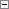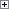This topic contains examples of how to use the type conversion rules for arithmetic operators. The examples illustrate how the operators should be used and the effect of the type conversion that the C/AL compiler performs. The examples have been divided into groups corresponding to the data types in C/AL.

Using Type Conversion Rules for Arithmetic Operators

For a full description of the type conversion rules in C/AL, see Type Conversion Rules for Arithmetic Operators, which provides a full description of all the possible uses of C/AL operators and the resulting data types.

Example 1

This table illustrates type conversion in integer operator expressions.

Operator Name Expression Resulting data type

+

time + integer

time

-

time difference

time - time

integer

The time unit is milliseconds. If time is undefined (0T), a run-time error will occur.

Example 2

This table illustrates type conversion in text and code (String) operator expressions.

Operator Name Expression Resulting data type

+

Concatenation

text + text

text

+

Concatenation

text + code

text

+

Concatenation

code + text

text

+

Concatenation

code + code

code